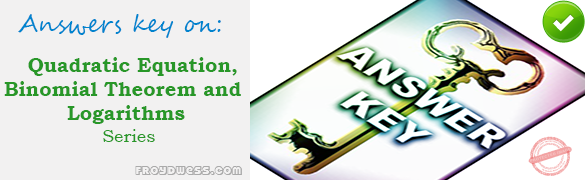# MCQ in Quadratic Equation, Binomial Theorem and Logarithms Part 2 – Answers

(Last Updated On: February 6, 2020)Below are the answers key for the Multiple Choice Questions in Quadratic Equation, Binomial Theorem and Logarithms Part 2.

51. B. 25

52. C. 3

53. D. 1

54. A. 4.19

55. C. 9

56. C. 2x + y – 1

57. C. (y log x)/ (x log y)

58. A. (1/a)(log P-b)

59. B. a2 log a

60. A. logb a

61. C. 6

62. A. 9

63. C. 6

64. B. 125

65. C. 3.47

66. C. 1/8n

67. C. y = x3

68. C. log7 (1/49) = -2

69. A. (log (x))/n

70. C. xy

71. B. log [ x/(y + z) ]

72. A. 9.9

73. A. 1

74. D. 3.761

75. A. 9

76. C. 8

77. A. 2

78. D. 3.79

79. D. complex number

80. C. -2.10

81. B. –(66339/128a11)

82. B. 126720

83. D. 5760×8

84. C. 25344×9

85. B. 5005

86. A. 256

87. D. 262 144

88. A. less than 2

89. B. 0

90. A. C/A

91. D. 26

92. C. 17/2

93. C. 1/u and 1/v

94. C. 2

95. A. 5×2 + 3x – 2 = 0

96. C. 12×2 – 7x + 1 = 0

97. B. 4

98. B. 4

99. A. 3

100. D. x2 – 3x – 6 = 0

### Online Questions and Answers in Quadratic Equation, Binomial Theorem and Logarithms Series

Quadratic Equation, Binomial Theorem and Logarithms MCQs
PART 1: MCQs from Number 1 – 50                        Answer key: PART I
PART 2: MCQs from Number 51 – 100                   Answer key: PART II

Please do Subscribe on YouTube!

P inoyBIX educates thousands of reviewers and students a day in preparation for their board examinations. Also provides professionals with materials for their lectures and practice exams. Help me go forward with the same spirit.

“Will you subscribe today via YOUTUBE?”

Subscribe